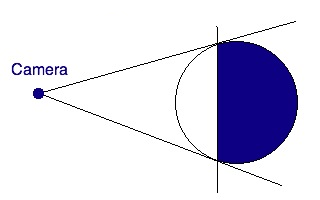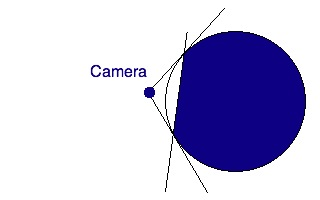Horizon Culling

# Horizon Culling

This past year I’ve been working on a fractal planet generator using C# and XNA. The system allows transitioning from space all the way down to ground level, and features accurately sized planets, atmospheric scattering shaders, oceans, water reflections, and other typical 3D features. There’s a lot of work to be done to take it where I want to go with it someday, and parts of it don’t work well at all, but it is a good proof of concept and I made huge leaps in understanding 3D graphics techniques. I don’t currently have plans to go over the entire system here, but I’ll pick out parts of it to discuss. And for this post I’ll start with horizon culling.

In 3D graphics, a technique that’s often used to improve performance is called culling. Culling means to reduce the population of a wild animal by selective slaughter. It also means, and this is the definition we’ll be using here, to select from a large quantity. For Horizon Culling, the large quantity we’ll be selecting from is the tens of thousands of triangles used to draw a spherical planet. And we’ll be using the horizon to determine which triangles to cull.

The image below shows why horizon culling is so useful. The camera is looking at the planet, and it can’t see what’s behind the horizon due to the curvature. The dark blue section shows the part of the planet that is invisible to the camera. Since the camera can’t see it, it makes no sense to draw it.The previous image shows that we can eliminate drawing more than half the planet. Now check out the following image. Note that the camera is much closer to the surface of the planet, which means that it can’t see nearly as far before the horizon interferes. This means we can eliminate drawing a huge portion of the planet.The closer you get to the surface of the planet, the less you have to draw. You can use that increased efficiency to draw more detail close to the camera where it matters.

When I was coming up with the algorithm to implement this in my planet generator, I developed a test Windows application that would let me visualize everything in the simplest way I could come up with. Over the next two or three posts I want to do a kind of tutorial and walk you through creating that app. When we’re all through you should have a pretty good idea how the horizon culling works and how to apply the calculations to your app if you’re geeky enough to be creating something to display full sized planets.

Most of the cool things in 3D graphics require math, and this is no exception, but let’s save the math for later. In the remainder of this post we’ll go through the preliminary step of creating a C# Vector class. Now, there’s already a Vector class in XNA that does everything we need, but I wanted the app to work without requiring XNA installation. You may want to read up on vectors if you’re not familiar.

Here’s the Vector class we’ll be using. It’s a basic implementation of only the things that we require in our app and leaves out some useful things such as a cross product. It should be fairly painless to add that sort of thing if you need it.

```public struct Vector
{
public double X; // in most cases you'd probably want to use single precision
public double Y; // floats here, but in my case I needed to be able to position
public double Z; // things at a solar system scale and single just doesn't cut it

public Vector(double X, double Y, double Z)
{
this.X = X;
this.Y = Y;
this.Z = Z;
}

public void SetValue(double X, double Y, double Z)
{
this.X = X;
this.Y = Y;
this.Z = Z;
}

public double Length()
{
return Math.Sqrt(X * X + Y * Y + Z * Z); // length of a 3D vector is sqrt(X^2 + Y^2 + Z^2)
}

public void Normalize()
{
double L = Math.Sqrt(X * X + Y * Y + Z * Z); // get the vector length
X /= L; // divide each component of the vector by the length
Y /= L; // so we end up with a vector of length 1
Z /= L;
}

public static double Dot(ref Vector V1, ref Vector V2)
{
return V1.X * V2.X + V1.Y * V2.Y + V1.Z * V2.Z;
}

public override string ToString()
{
return "&lt;" + X.ToString() + "," + Y.ToString() + "," + Z.ToString() + "&gt;";
}
}
```

You may wonder why this is a struct instead of a class. A struct in C# looks and acts enough like a class that I feel free to call it a class, even though I suppose technically it isn’t. I’ve not really studied into it that much. One big difference though is that structs don’t cause garbage collection because they’re allocated on the stack. Garbage collection is bad in 3D graphics applications. Another way to look at this is that structs act like much like a value (e.g. an int) rather than a reference (e.g. a class, which is in effect a pointer).

I think it’s time to bring this post to a close. In my next couple of posts I’ll go over the details of the culling algorithm and the rest of the app. When we’re all done I’ll post the entire app.